Function Repository Resource:

# MultispacePlot3D

Plot multispace in 3D

Contributed by: Nikolay Murzin
 ResourceFunction["MultispacePlot3D"][mwf] returns a visualization of multispace for multiway function mwf. ResourceFunction["MultispacePlot3D"][mwf,prop] returns the property prop involved in computing a visualization of multispace.

## Details and Options

Property prop may be one of following:
 "RawGraphs" return a list of 2D graphs without computing a 3D embedding "Association" an Association returned by an underlying algorithm "Graph" GraphPlot3D of a multispace "View" Graphics3D with convenient controls (default)
Options may include any option for GraphPlot3D or the resource functions MultiPerspectiveEmbedding and MultiPerspectiveEmbeddingViewer. ResourceFunction["MultispacePlot3D"] also accepts the following options:
 "ObjectType" "State" "State" or "Event" multispace "InfiniteDistanceIncrement" 1 additional distance between disconnected vertices "DistanceMatrixWeights" Automatic vector of coefficients with which to multiply each distance matrix Method "MPSE" algorithm to use (only one is currently supported)

## Examples

### Basic Examples (4)

Return raw graphs of a multiway system:

 In:=Out=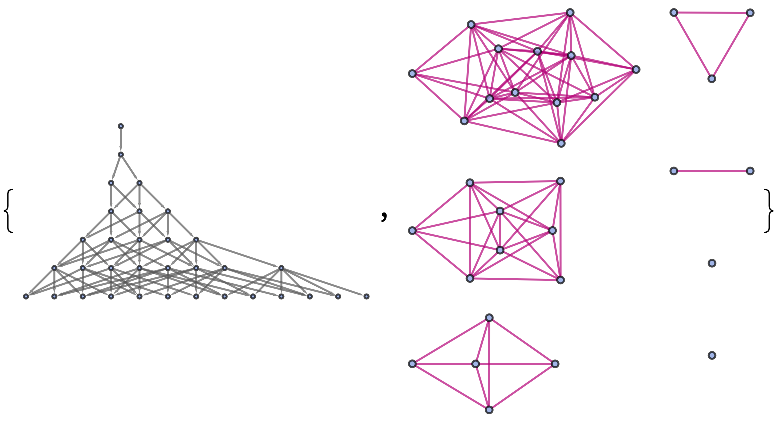Return an Association with the results of an embedding algorithm:

 In:=Out=Return 3D graphics for a resulting Graph:

 In:=Out=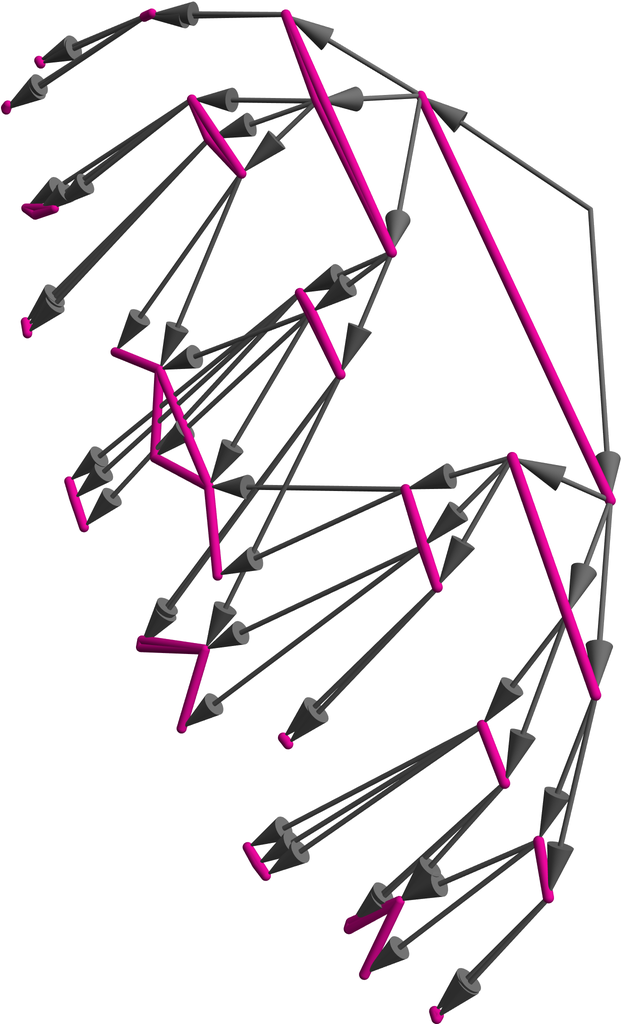Add multiperspective controls to view the resulting Graph:

 In:=Out=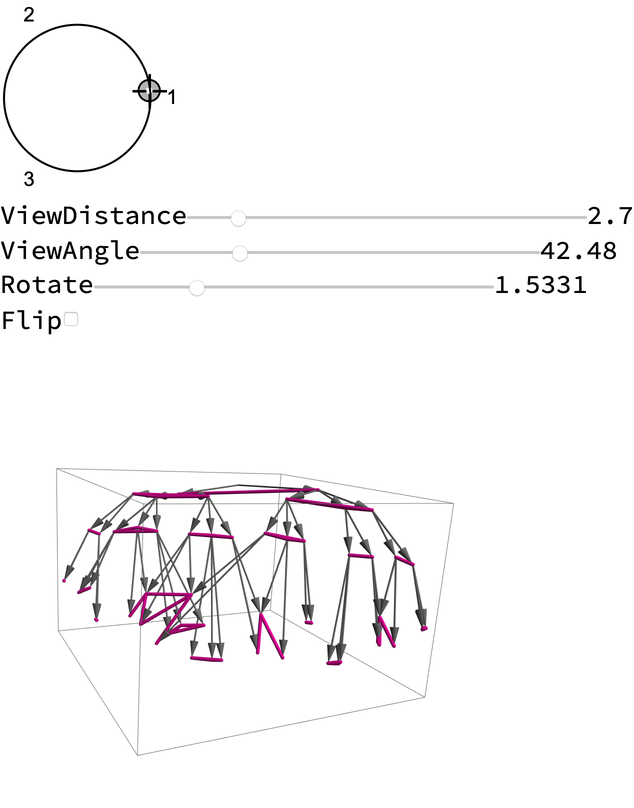### Options (4)

#### ObjectType (2)

Plot the multispace of a Turing machine for events:

 In:=Out=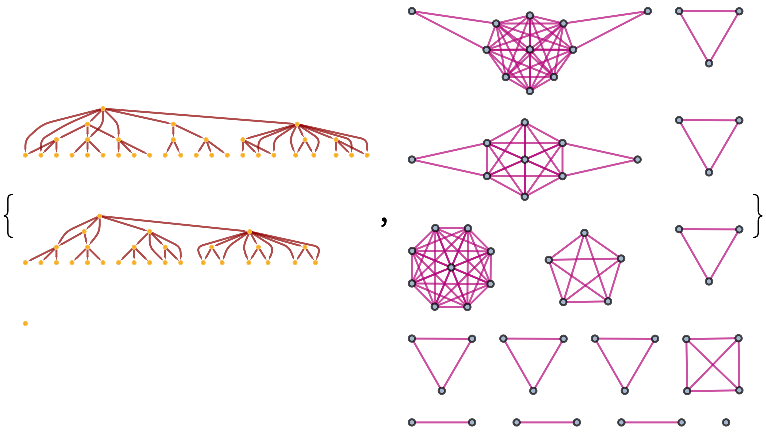Or in 3D:

 In:=Out=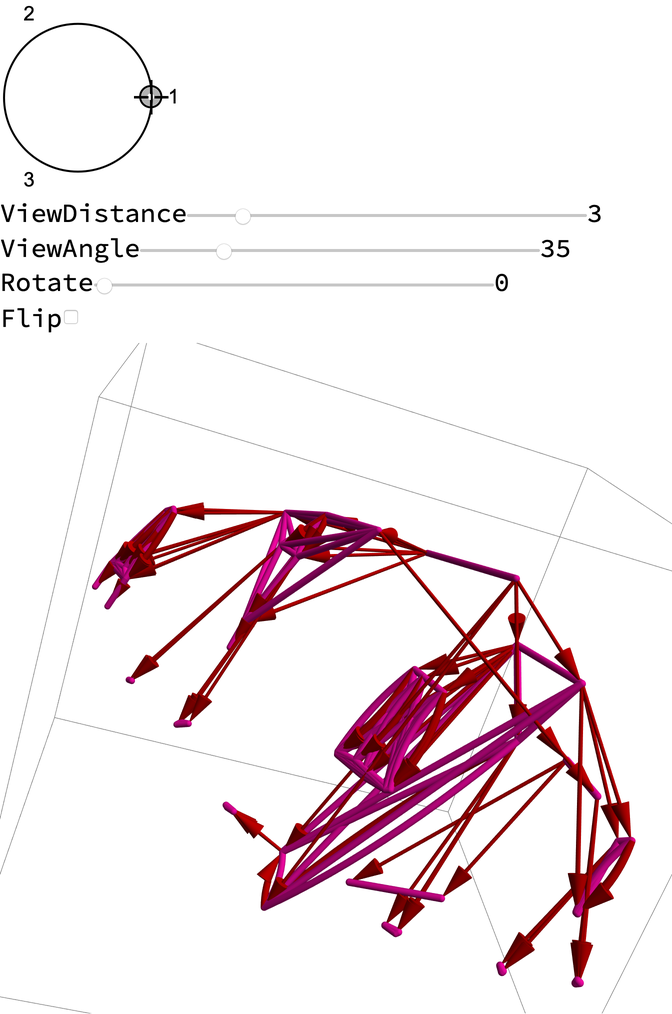#### InfiniteDistanceIncrement (1)

Increase distance between branchially disconnected states:

 In:=Out=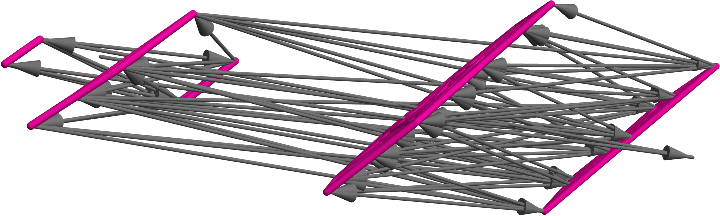#### DistanceMatrixWeights (1)

Weights are constant multipliers of distances after normalization. In order, they are {time, space, branchial space}:

 In:=Out=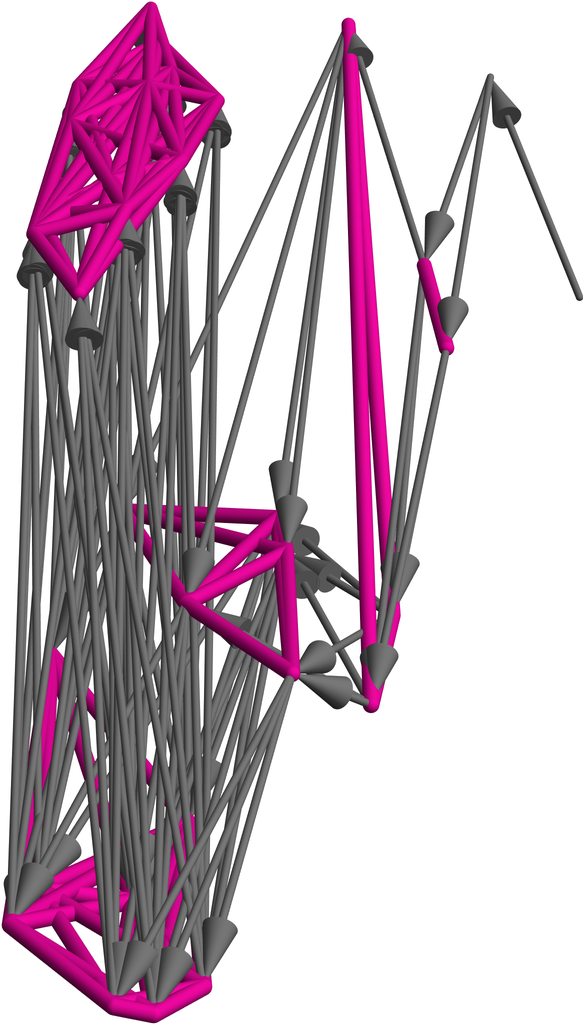### Scope (2)

Options can be passed to the underlying algorithm:

 In:=Out=Or to the resulting Graphics3D object:

 In:=Out=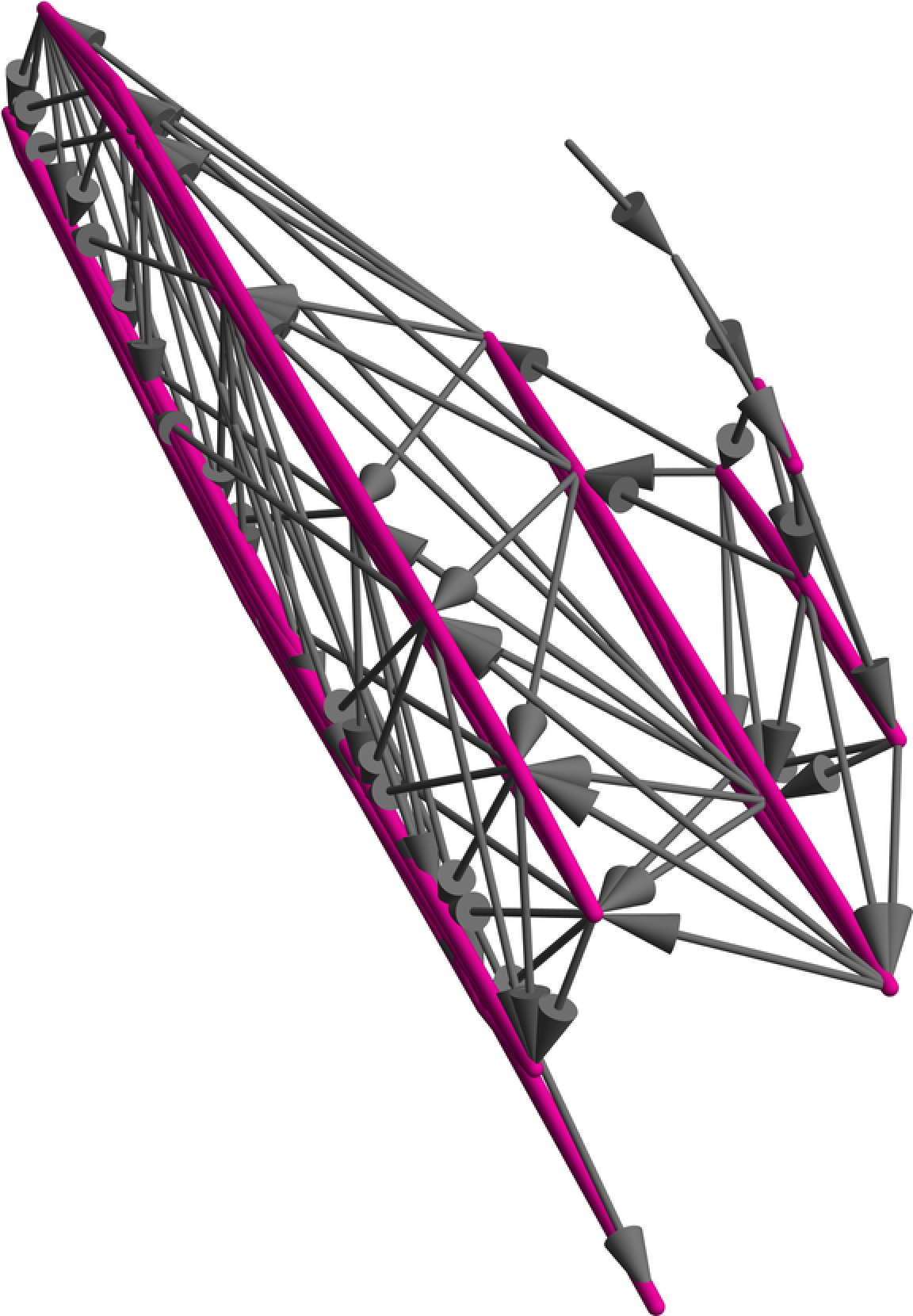N. Murzin

## Version History

• 1.0.1 – 09 August 2021
• 1.0.0 – 17 July 2020